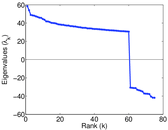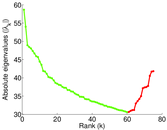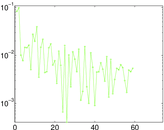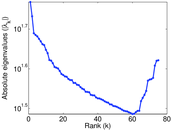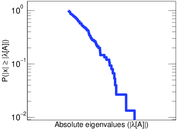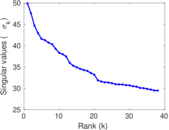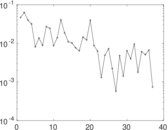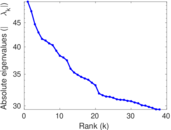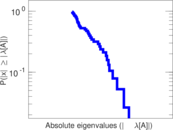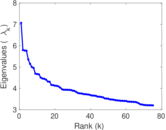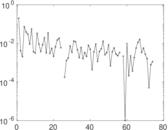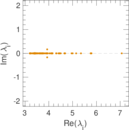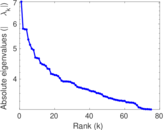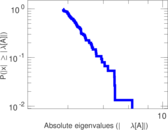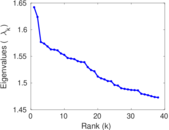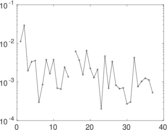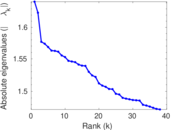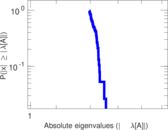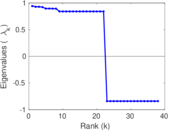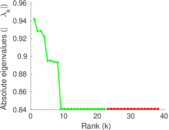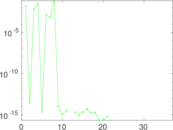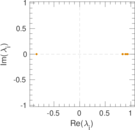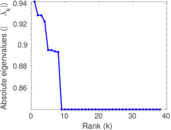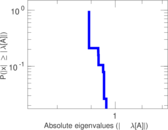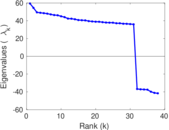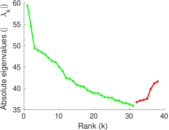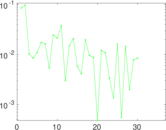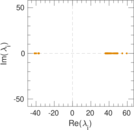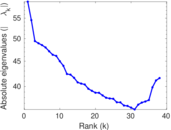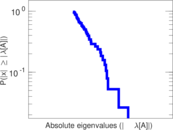# CiteSeer

This is the citation network extracted from the CiteSeer digital library. Nodes are publications and the directed edges denote citations. Publications can cite themselves in this dataset, and therefore the network includes loops. The network was extracted by hand by Jérôme Kunegis before the start of the KONECT project, and therefore no extraction code remains.

 Code `CS` Internal name `citeseer` Name CiteSeer Data source http://citeseer.ist.psu.edu/oai.html AvailabilityDataset is available for download Consistency checkDataset passed all tests Category Citation network Node meaning Publication Edge meaning Citation Network format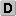Unipartite, directed Edge typeUnweighted, no multiple edges ReciprocalContains reciprocal edges Directed cyclesContains directed cycles Loops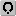Contains loops

## Statistics

 Size n = 384,413 Volume m = 1,751,463 Loop count l = 6,873 Wedge count s = 81,727,579 Claw count z = 9,032,698,085 Cross count x = 1,888,767,722,244 Triangle count t = 1,351,820 Square count q = 25,382,500 4-Tour count T4 = 533,442,606 Maximum degree dmax = 1,739 Maximum outdegree d+max = 289 Maximum indegree d−max = 1,739 Average degree d = 9.112 40 Fill p = 1.185 24 × 10−5 Size of LCC N = 365,154 Size of LSCC Ns = 16,208 Relative size of LSCC Nrs = 0.042 163 0 Diameter δ = 34 50-Percentile effective diameter δ0.5 = 5.645 46 90-Percentile effective diameter δ0.9 = 7.957 12 Median distance δM = 6 Mean distance δm = 6.348 17 Gini coefficient G = 0.578 549 Balanced inequality ratio P = 0.284 825 Outdegree balanced inequality ratio P+ = 0.321 688 Indegree balanced inequality ratio P− = 0.237 567 Relative edge distribution entropy Her = 0.945 199 Power law exponent γ = 1.634 33 Tail power law exponent γt = 2.731 00 Tail power law exponent with p γ3 = 2.731 00 p-value p = 0.000 00 Outdegree tail power law exponent with p γ3,o = 4.061 00 Outdegree p-value po = 0.371 000 Indegree tail power law exponent with p γ3,i = 2.691 00 Indegree p-value pi = 0.000 00 Degree assortativity ρ = −0.061 826 4 Degree assortativity p-value pρ = 0.000 00 Clustering coefficient c = 0.049 621 7 Operator 2-norm ν = 49.521 6 Cyclic eigenvalue π = 7.065 03 Algebraic connectivity a = 0.004 605 12 Spectral separation |λ1[A] / λ2[A]| = 1.086 33 Reciprocity y = 0.013 567 5 Non-bipartivity bA = 0.286 994 Normalized non-bipartivity bN = 0.004 807 89 Spectral bipartite frustration bK = 0.000 262 567 Controllability C = 202,338 Relative controllability Cr = 0.526 356

## Plots

### Fruchterman–Reingold graph drawing### Degree distribution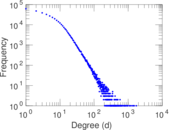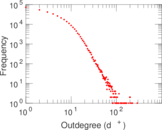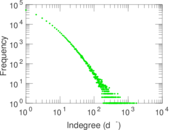### Cumulative degree distribution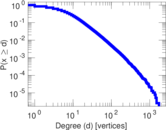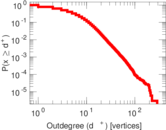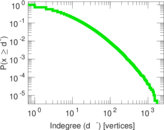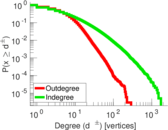### Lorenz curve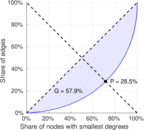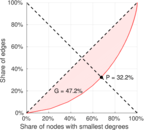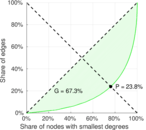### Spectral distribution of the adjacency matrix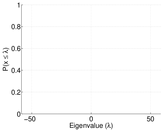### Spectral distribution of the normalized adjacency matrix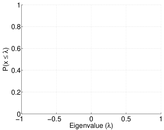### Spectral distribution of the Laplacian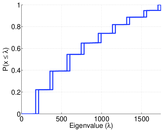### Spectral graph drawing based on the adjacency matrix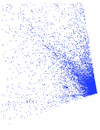### Spectral graph drawing based on the Laplacian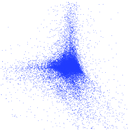### Spectral graph drawing based on the normalized adjacency matrix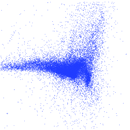### Degree assortativity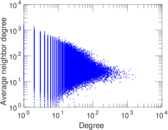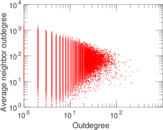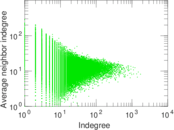### Zipf plot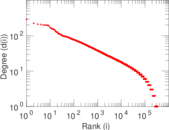### Hop distribution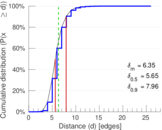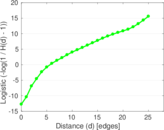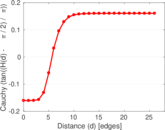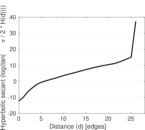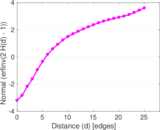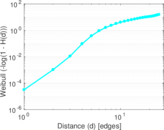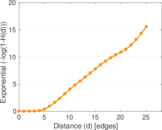### Delaunay graph drawing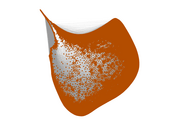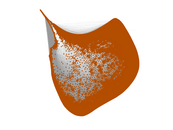### In/outdegree scatter plot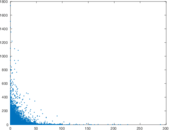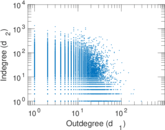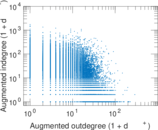### Clustering coefficient distribution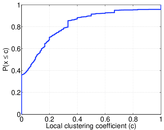### SynGraphy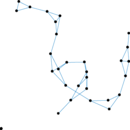### Matrix decompositions plots